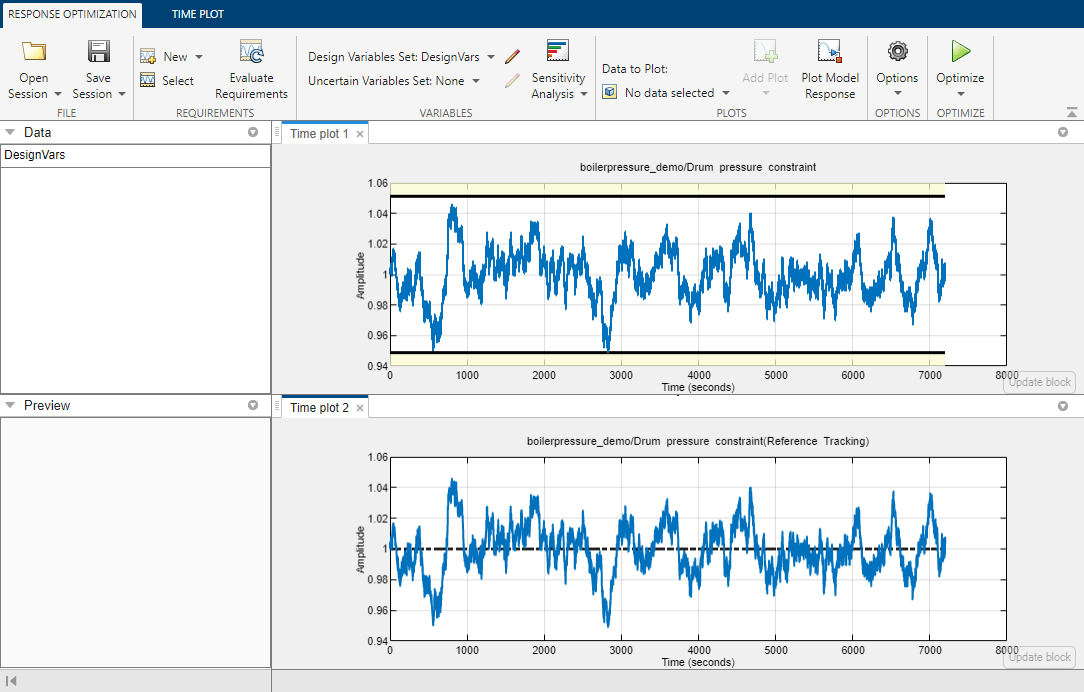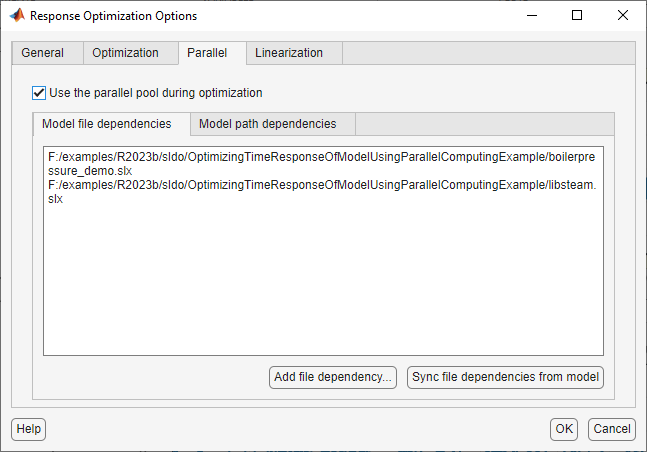# Optimizing Time-Domain Response of Simulink Models Using Parallel Computing

This example shows how to use parallel computing to optimize the time-domain response of a Simulink® model. You use Simulink Design Optimization™ and Parallel Computing Toolbox™ to tune the gains of a discrete PI controller of a boiler to meet the design requirements. The example also shows how the software automatically handles model file dependencies.

This example requires Parallel Computing Toolbox.

### Opening the Model

The Simulink model consists of a boiler model and a discrete PI controller. When using parallel computing, Simulink Design Optimization performs a model dependency check, which recognizes the boiler model library as an installed library.

```open_system('boilerpressure_demo') ```### Design Requirements

The boiler pressure is regulated by a discrete PI controller. The design requirement for the controller is to limit the pressure variation of the boiler within +-%5 of the nominal pressure.

The initial controller has fairly good regulation characteristics but in the presence of additional heat disturbances, modeled by the Heat Disturbance block, we want to tune the controller performance to provide tighter pressure regulation.

Double-click the 'Response Optimization GUI with preloaded data' block in the Simulink model to open a pre-configured Response Optimizer session. The Response Optimizer is configured with:

1. Upper and lower bounds representing a +-5% allowable range on the drum pressure

2. A reference tracking objective to minimize the deviation of the drum pressure from nominal

3. The PI controller gains, Kp and Ki, are selected for tuning

Click Plot Model Response to display the drum pressure variations with the initial controller.### Configuring and Running the Optimization in the GUI Using Parallel Computing

When computing the model response with the initial controller, this complex model took a long time to simulate. Using parallel computing can reduce the optimization time by simulating the model in parallel. For more information on parallel computing and optimization performance see the tutorial Improving Optimization Performance Using Parallel Computing.

To configure the optimization problem to use parallel computing click Options in the Response Optimizer and select the Parallel Options tab. Select the "Use the parallel pool during optimization" option. This triggers an automated search for any model dependencies. In this example, the Steam table library (`libsteam.slx`) is found as a model dependency (in addition to the `boilerpressure_demo` model itself), and is displayed in the Model file dependencies list box.Clicking OK configures the optimization to use parallel computing.

To run the optimization click the Optimize button. A progress window opens displaying optimization progress and the plots update to show the optimized response.The final response shows that the optimized regulator tracks the reference pressure much more closely and the drum pressure constraints are satisfied.

### Configuring and Running the Optimization at the Command Line

You can also use the command line functions to configure the optimization to use parallel computing and run the optimization.

Select the model variables for optimization and set lower limits

```p = sdo.getParameterFromModel('boilerpressure_demo',{'Kp','Ki'}); p(1).Minimum = 0.001; p(2).Minimum = 0.001; ```

Select the model signal to bound and create a simulator to simulate the model.

```nPressure = Simulink.SimulationData.SignalLoggingInfo; nPressure.BlockPath = 'boilerpressure_demo/1//y0'; nPressure.OutputPortIndex = 1; nPressure.LoggingInfo.NameMode = 1; nPressure.LoggingInfo.LoggingName = 'nPressure'; simulator = sdo.SimulationTest('boilerpressure_demo'); simulator.LoggingInfo.Signals = nPressure; ```

Get the optimization requirements defined by the check blocks in the model so that we can use them in the optimization problem.

```bnds = getbounds('boilerpressure_demo/Drum pressure constraint'); PressureLimits = [bnds{:}]; bnds = getbounds('boilerpressure_demo/Drum pressure constraint(Reference Tracking)'); PressureRegulation = [bnds{:}]; requirements = struct(... 'PressureLimits', PressureLimits, ... 'PressureRegulation', PressureRegulation); ```

Define the function called during optimization. Notice that the function uses the simulator and requirements defined earlier to evaluate the design.

```evalDesign = @(p) boilerpressure_design(p,simulator,requirements); type boilerpressure_design ```
```function design = boilerpressure_design(p,simulator,requirements) %BOILERPRESSURE_DESIGN % % The boilerpressure_design function is used to evaluate a boiler % controller design design. % % The |p| input argument is the vector of controller parameters. % % The |simulator| input argument is a sdo.SimulinkTest object used to % simulate the |boilerpressure_demo| model and log simulation signals. % % The |requirements| input argument contains the design requirements used % to evaluate the boiler controller design. % % The |design| return argument contains information about the design % evaluation that can be used by the |sdo.optimize| function to optimize % the design. % % see also sdo.optimize, sdoExampleCostFunction % Copyright 2011 The MathWorks, Inc. %% Simulate the model % % Use the simulator input argument to simulate the model and log model % signals. % % First ensure that we simulate the model with the parameter values chosen % by the optimizer. % simulator.Parameters = p; %% % Simulate the model and log signals. % simulator = sim(simulator); %% % Get the simulation signal log, the simulation log name is defined by the % model |SignalLoggingName| property % logName = get_param('boilerpressure_demo','SignalLoggingName'); simLog = get(simulator.LoggedData,logName); %% Evaluate the design requirements % % Use the requirements input argument to evaluate the design requirements % % Check the Pressure signal against the |PressureLimits| requirements. % nPressure = get(simLog,'nPressure'); c = [... evalRequirement(requirements.PressureLimits(1),nPressure.Values); ... evalRequirement(requirements.PressureLimits(2),nPressure.Values)]; %% % Use the PressureLimits requirements as non-linear constraints for % optimization. design.Cleq = c(:); %% % Check the pressure signal against the |PressureRegulation| requirement. % f = evalRequirement(requirements.PressureRegulation,nPressure.Values); %% % Use the PressureRegulation requirement as an objective for optimization. design.F = f; end ```

Setup optimization options to use the parallel pool and specify the model and model files dependencies.

```opt = sdo.OptimizeOptions; opt.UseParallel = true; opt.OptimizedModel = 'boilerpressure_demo'; [dirs,files] = sdo.getModelDependencies('boilerpressure_demo'); opt.ParallelFileDependencies = files; ```

To run the optimization using the parallel pool pass the optimization options, `opt`, to the `sdo.optimize` command.Close the model.

```bdclose('boilerpressure_demo') ```